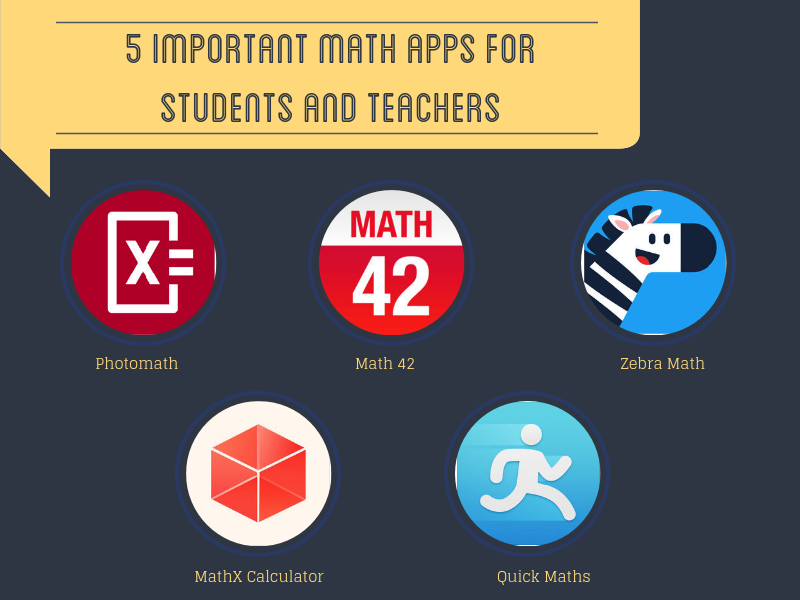# 5 Good Educational Math Apps for Students

In today’s post we are sharing with you 5 math apps that are featured today in Apple’s New and Notable category in iTunes App Store. Some of you are probably already familiar with Photomath and Math 42 for we have already covered them in multiple instances here in the past. Students can draw on them to get help with their math homework. The three remaining apps (Quick Maths, MathX, and Zebra Math) are featured here for the first time. You may want to try them out and see if they can be of any help for your students.1- Photomath
‘Simply point your camera toward a math problem and Photomath will magically show the result with a detailed step-by-step instructions…Photomath supports arithmetics, integers, fractions, decimal numbers, roots, algebraic expressions, linear equations/inequalities, quadratic equations/inequalities, absolute equations/inequalities, systems of equations, logarithms, trigonometry, exponential and logarithmic functions, derivatives and integrals.’

2- Math 42
‘Math 42 helps over 2.200.000 middle-school, high-school and college students, to solve their math problems and get better grades at the fraction of the cost of a private tutor. MATH 42 helps with (1) intelligent approaches to the solution, (2) step-by-step solutions of their problems (3) an Assessment Center.’

3- Zebra Math
‘Zebra Math brings the amazing math learning experience. The levels and tasks help children learn math step by step, which have been proved to be systematic and effective. Enjoy exploring Zebra Math.’

4- MathX
‘MathX brings the power of a graphic and scientific calculator on your iPhone and iPad. It can solve more than 18 operations and gives you step-by-step explanations. It allows you to graph and compare up to seven functions simultaneously.’

5- Quick Maths
‘Quick Maths is perfect for students in grades 2 to 6, or for anyone wanting to improve their general maths skills. With multiple difficulty levels and a focus on self-improvement, Quick Maths provides increasing challenges as your skills develop.’